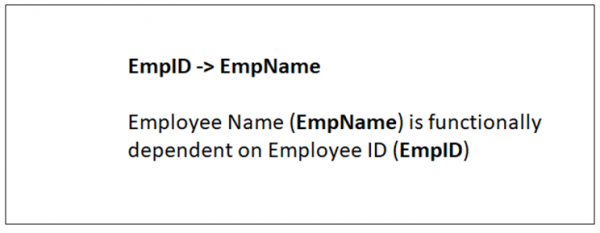# Types of dependencies in DBMS

Dependencies in DBMS is a relation between two or more attributes. It has the following types in DBMS −

• Functional Dependency
• Fully-Functional Dependency
• Transitive Dependency
• Multivalued Dependency
• Partial Dependency

## Functional Dependency

If the information stored in a table can uniquely determine another information in the same table, then it is called Functional Dependency. Consider it as an association between two attributes of the same relation.

If P functionally determines Q, then

 P -> Q

Let us see an example −

<Employee>

 EmpID EmpName EmpAge E01 Amit 28 E02 Rohit 31

In the above table, EmpName is functionally dependent on EmpID because EmpName can take only one value for the given value of EmpID:

 EmpID -> EmpName

The same is displayed below −## Fully-functionally Dependency

An attribute is fully functional dependent on another attribute, if it is Functionally Dependent on that attribute and not on any of its proper subset.

For example, an attribute Q is fully functional dependent on another attribute P, if it is Functionally Dependent on P and not on any of the proper subset of P.

Let us see an example −

<ProjectCost>

 ProjectID ProjectCost 001 1000 002 5000

<EmployeeProject>

 EmpID ProjectID Days (spent on the project) E099 001 320 E056 002 190

The above relations states:

 EmpID, ProjectID, ProjectCost -> Days

However, it is not fully functional dependent.

Whereas the subset {EmpID, ProjectID} can easily determine the {Days} spent on the project by the employee.

This summarizes and gives our fully functional dependency −

 {EmpID, ProjectID}  -> (Days)

## Transitive Dependency

When an indirect relationship causes functional dependency it is called Transitive Dependency.

If  P -> Q and Q -> R is true, then P-> R is a transitive dependency.

## Multivalued Dependency

When existence of one or more rows in a table implies one or more other rows in the same table, then the Multi-valued dependencies occur.

If a table has attributes P, Q and R, then Q and R are multi-valued facts of P.

It is represented by double arrow −

 ->->

For our example:

 P->->QQ->->R

In the above case, Multivalued Dependency exists only if Q and R are independent attributes.

## Partial Dependency

Partial Dependency occurs when a nonprime attribute is functionally dependent on part of a candidate key.

The 2nd Normal Form (2NF) eliminates the Partial Dependency. Let us see an example −

<StudentProject>

 StudentID ProjectNo StudentName ProjectName S01 199 Katie Geo Location S02 120 Ollie Cluster Exploration

In the above table, we have partial dependency; let us see how −

The prime key attributes are StudentID and ProjectNo.

As stated, the non-prime attributes i.e. StudentName and ProjectName should be functionally dependent on part of a candidate key, to be Partial Dependent.

The StudentName can be determined by StudentID that makes the relation Partial Dependent.

The ProjectName can be determined by ProjectID, which that the relation Partial Dependent.